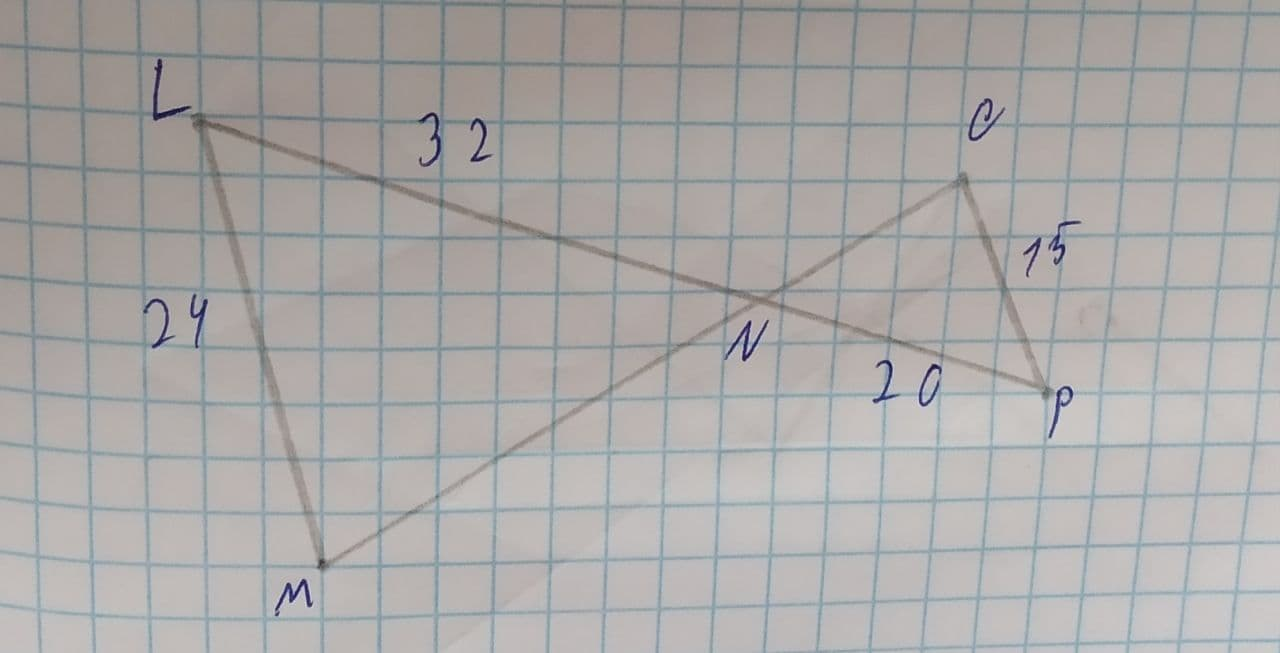# Determine whether the triangles are similar. If similar, state how (AA\sim, SSS\sim,\ or\ SAS\sim), and write a similarity statement.Caelan 2021-08-05 Answered
Determine whether the triangles are similar. If similar, state how (), and write a similarity statement.You can still ask an expert for help

• Questions are typically answered in as fast as 30 minutes

Solve your problem for the price of one coffee

• Math expert for every subject
• Pay only if we can solve itDerrick
In :
$\frac{LM}{PO}=\frac{LN}{PN}$
$\frac{24}{15}=\frac{32}{20}$
$=\frac{8}{5}=\frac{8}{5}$
$\mathrm{\angle }LNM=\mathrm{\angle }PNO$ (Vertically opposite angle)
(By SAS similarity)